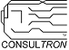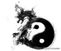PSoC™ 6Level 9Level 9

# Issue with HAL PWM code: When cyhal_pwm_set_duty_cycle(&pwm_obj, dc, frequency) where dc = 100%, pwm = 0%

I was using HAL PWM code to set the PWM resource to 100% dutycycle.  What I got is that at 100% dutycycle, the PWM turned OFF (effectively 0%).

The issue is actually in the function cyhal_pwm_set_period_and_compare().

This is because:

`cyhal_pwm_set_duty_cycle(&obj, 100, frequencyhal_hz){...     uint32_t period = obj->clock_hz / frequencyhal_hz;     uint32_t width = (uint32_t)(100 * 0.01f * period);     cyhal_pwm_set_period_and_compare(obj, period, width);     /* Here period = width @ 100% dc */     {     ...          Cy_TCPWM_PWM_SetPeriod0(obj->base, obj->resource.channel_num, period - 1u);     /* The period count is corrected here */     ...     else     {          Cy_TCPWM_PWM_SetCompare0(obj->base, obj->resource.channel_num, compare);                    /* Although the period was corrected (period-1) the compare in this case is period + 1.  The compare count was not corrected. */               /* When compare > period, the result is an effective 0% dc */     }`

May I recommend the following changes to function cyhal_pwm_set_period_and_compare():

`static cy_rslt_t cyhal_pwm_set_period_and_compare(cyhal_pwm_t *obj, uint32_t period, uint32_t compare){    if (period < 1 || period > (uint32_t)((1 << CYHAL_TCPWM_DATA[obj->resource.block_num].max_count)) - 1)        return CYHAL_PWM_RSLT_BAD_ARGUMENT;    period = period - 1u;    /* Make the period count correction here. */                            /* This fixes the issue where period == compare (ie duty_cycle == 100 percent) */    if (compare > period)        compare = period;    cyhal_gpio_t pin = obj->pin;    cyhal_gpio_t pin_compl = obj->pin_compl;    Cy_TCPWM_PWM_SetCompare0(obj->base, obj->resource.channel_num, 0u);    Cy_TCPWM_PWM_SetPeriod0(obj->base, obj->resource.channel_num, period);    bool swapped_pins = (CY_UTILS_GET_RESOURCE(pin, cyhal_pin_map_tcpwm_line_compl) != NULL) && (CY_UTILS_GET_RESOURCE(pin_compl, cyhal_pin_map_tcpwm_line) != NULL);    bool is_center_aligned = (TCPWM_CNT_TR_CTRL2(obj->base, obj->resource.channel_num) == CY_TCPWM_PWM_MODE_CNTR_OR_ASYMM) ||                                (TCPWM_CNT_TR_CTRL2(obj->base, obj->resource.channel_num) == CY_TCPWM_PWM_MODE_CNTR_OR_ASYMM_UO_SWAPPED);    if ((swapped_pins) && (!is_center_aligned))    {        Cy_TCPWM_PWM_SetCompare0(obj->base, obj->resource.channel_num, period - compare);    }    else    {        Cy_TCPWM_PWM_SetCompare0(obj->base, obj->resource.channel_num, compare);    }    return CY_RSLT_SUCCESS;}`

Len

Len
"Engineering is an Art. The Art of Compromise."
2 RepliesModeratorModerator

# Re: Issue with HAL PWM code: When cyhal_pwm_set_duty_cycle(&pwm_obj, dc, frequency) where dc = 100%, pwm = 0%

Hello Len,

Thank you for pointing this out. The fix has been added and you should see it in the next release of the psoc6hal​.

Regards,

DheerajLevel 3Level 3

# Re: Issue with HAL PWM code: When cyhal_pwm_set_duty_cycle(&pwm_obj, dc, frequency) where dc = 100%, pwm = 0%

It seems that the new version still has problems...

I reopen a topic: [Problem] PWM duty setting issue by (PWM-HAL)Top labels